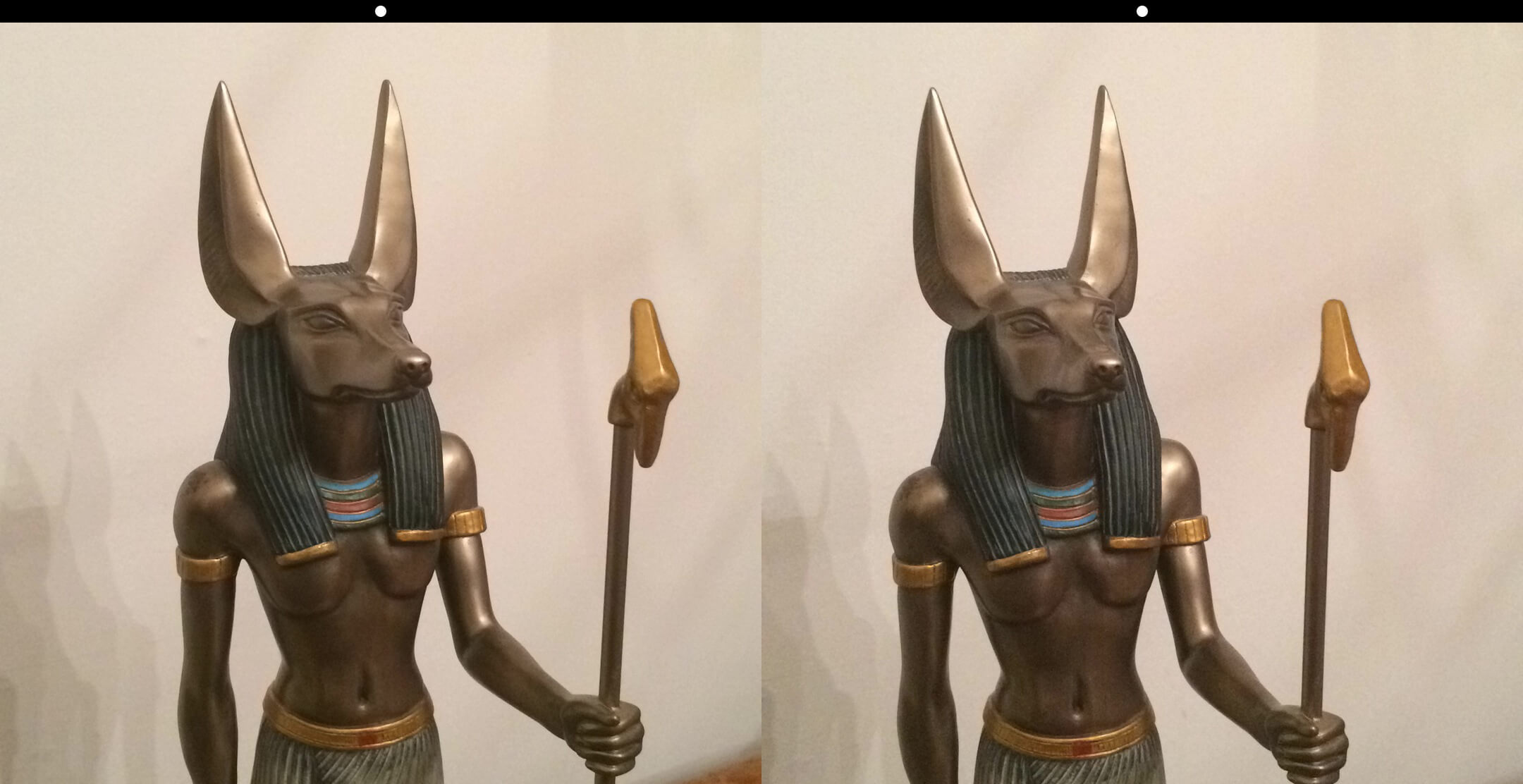## 数据存储

### 位图

GIF 和 PNG 对于具有大面积相同颜色的图像是最好的选择，因为它们使用的 (主要是基于游程长度编码的) 压缩算法可以减少存储需求。这种压缩是无损的，这意味着图像质量不会被压缩过程影响。

## 用程序操作图像

``````-(UIImage*)composeStereogramLeft:(UIImage *)leftImage right:(UIImage *)rightImage
{
float w = leftImage.size.width;
float h = leftImage.size.height;
UIGraphicsBeginImageContext(CGSizeMake(w * 2.0, h + 32.0));
[leftImage drawAtPoint:CGPointMake(0.0, 32.0)];
[rightImage drawAtPoint:CGPointMake(w, 32.0)];
float leftCircleX = (w / 2.0) - 8.0;
float rightCircleX = leftCircleX + w;
float circleY = 8.0;
[[UIColor blackColor] setFill];
UIRectFill(CGRectMake(0.0, 0.0, w * 2.0, 32.0));

[[UIColor whiteColor] setFill];
CGRect leftRect = CGRectMake(leftCircleX, circleY, 16.0, 16.0);
CGRect rightRect = CGRectMake(rightCircleX, circleY, 16.0, 16.0);
UIBezierPath *path = [UIBezierPath bezierPathWithOvalInRect:leftRect];
[path appendPath:[UIBezierPath bezierPathWithOvalInRect:rightRect]];
[path fill];
UIImage *savedImg = UIGraphicsGetImageFromCurrentImageContext();
UIGraphicsEndImageContext();
return savedImg;
}
````````````UInt8 *rightPtr = rightBitmap;
UInt8 *leftPtr = leftBitmap;
UInt8 r1, g1, b1;
UInt8 r2, g2, b2;
UInt8 ra, ga, ba;

for (NSUInteger idx = 0; idx < bitmapByteCount; idx += 4) {
r1 = rightPtr; g1 = rightPtr; b1 = rightPtr;
r2 = leftPtr; g2 = leftPtr; b2 = leftPtr;

// r1/g1/b1 右侧图像，用于计算合并值
// r2/g2/b2 左侧图像，用于被合并值赋值
// ra/ga/ba 合并后的像素

ra = 0.7 * g1 + 0.3 * b1;
ga = b2;
ba = b2;

leftPtr = ra;
leftPtr = ga;
leftPtr = ba;
rightPtr += 4; // 指向下一个像素 (4字节, 包括透明度 alpha 值)
leftPtr += 4;
}

CGImageRef composedImage = CGBitmapContextCreateImage(_leftContext);
UIImage *retval = [UIImage imageWithCGImage:composedImage];
CGImageRelease(composedImage);
return retval;
``````